Home > CCA2 > Chapter 10 > Lesson 10.2.1 > Problem10-95

10-95.
1. Solve each equation. Homework Help ✎

1. log5(2x) = 3

2. log5(x + 1) = −1

3. log(4) − log(x) = 2

4. 2log3(6) + log3(y) = 4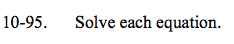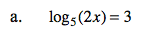53 = 2x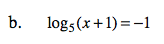5−1 = x + 1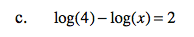$\log_{10}\Big(\frac{4}{x}\Big)=2$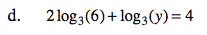log3(62) + log3(y) = 4